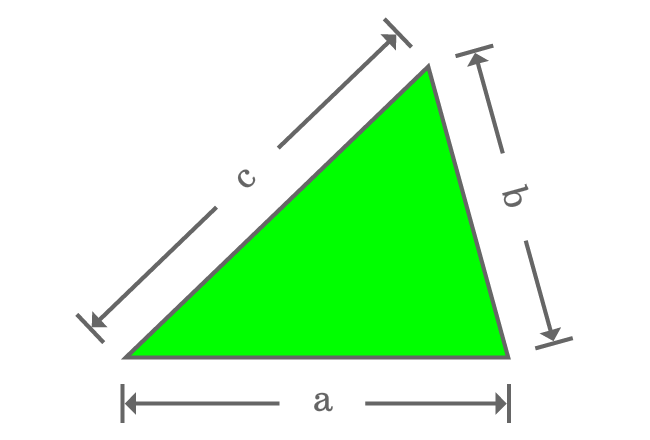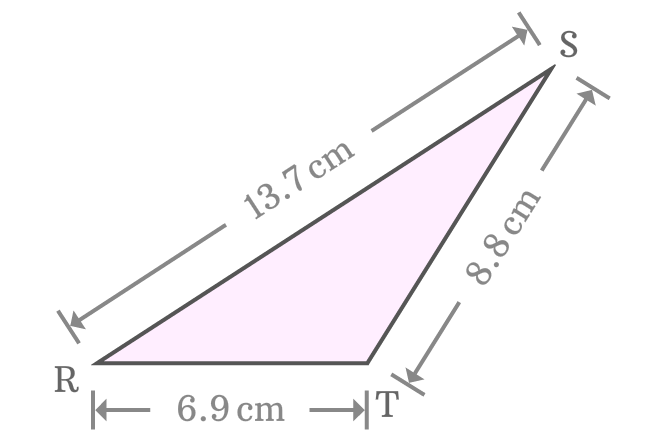# Perimeter of a triangle

The sum of lengths of all three sides of a triangle is called perimeter of a triangle.

## IntroductionThe boundary of a triangle is the perimeter of the triangle but its boundary is geometrically formed by the intersection of three line segments. Therefore, the perimeter of triangle is the total of the lengths of three sides of the triangle.

### Formula

If $a$, $b$ and $c$ are lengths of sides of a triangle, then the perimeter of the triangle is equal to sum of them.

$P_{t} \,=\, a+b+c$

Note, the letter $P_{t}$ is used in the formula to represent the perimeter of the triangle.

#### Example

$\Delta TRS$ is a triangle and we have to find the perimeter of this triangle.The length of each side of this triangle is given as follows

1. The length of side ($\small \overline{RT}$) is equal to $6.9 \, cm$
2. The length of side ($\small \overline{RS}$) is equal to $13.7 \, cm$
3. The length of side ($\small \overline{ST}$) is equal to $8.8 \, cm$

Now, it is time to find the perimeter for the given lengths of the triangle.

$P_{t} \,=\, RT+RS+ST$

$\implies P_{t} \,=\, 6.9+13.7+8.8$

$\,\,\, \therefore \,\,\,\,\,\, P_{t} \,=\, 29.4$

Therefore, it is calculated that the perimeter of the $\Delta TRS$ is $29.4 \, cm$. You can calculate the perimeter of any triangle in this way.

Latest Math Topics
Jun 26, 2023
Jun 23, 2023

Latest Math Problems
Jul 01, 2023
Jun 25, 2023
###### Math Questions

The math problems with solutions to learn how to solve a problem.

Learn solutions

Practice now

###### Math Videos

The math videos tutorials with visual graphics to learn every concept.

Watch now

###### Subscribe us

Get the latest math updates from the Math Doubts by subscribing us.